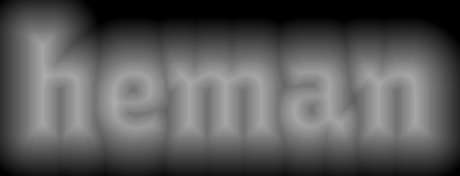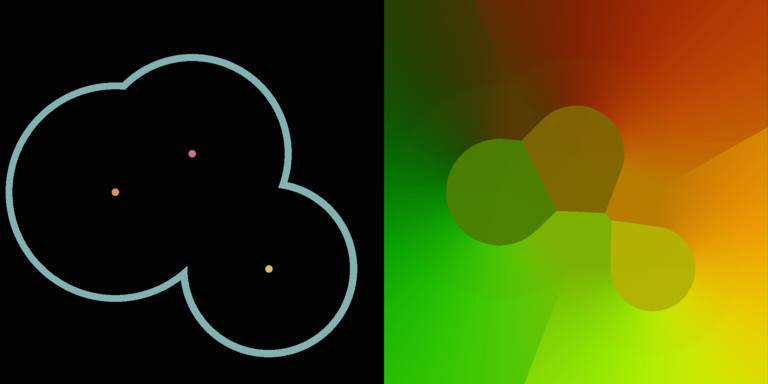# Distance Fields¶

All functions with the `heman_distance_` prefix are meant for creating distance fields. This is also known as a Euclidean Distance Transform.

Heman can also create a closest point coordinate field, which is like a distance field except that it encodes the ST of the nearest seed pixel. This can be used to create Voronoi diagrams or pick sheets.

## API¶

```// Create a one-band "signed distance field" based on the given input, using
// the fast algorithm described in Felzenszwalb 2012.
heman_image* heman_distance_create_sdf(heman_image* monochrome);

// Create a two-band "closest point coordinate field" containing the
// non-normalized texture coordinates of the nearest seed.  The result is
// related to the distance field but has a greater amount of information.
heman_image* heman_distance_create_cpcf(heman_image* seed);
```

## SDF Example¶

Here’s an example of a starting image (the “seed”) and its resulting signed distance field (SDF).The above image was generated with the following program:

 ``` 1 2 3 4 5 6 7 8 9 10 11 12 13 14``` ```#include #include "hut.h" #define OUTFOLDER "build/" #define INFOLDER "test/" int main(int argc, char** argv) { heman_image* seed = hut_read_image(INFOLDER "sdfseed.png", 1); heman_image* sdf = heman_distance_create_sdf(seed); heman_image_destroy(seed); hut_write_image(OUTFOLDER "sdfresult.png", sdf, -0.1, 0.1); heman_image_destroy(sdf); } ```

## CF Example¶

Here’s an example of a starting image (the “seed”) and its resulting CPCF.